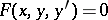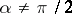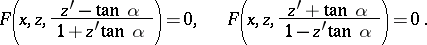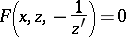# Isogonal trajectory

(diff) ← Older revision | Latest revision (diff) | Newer revision → (diff)

A plane curve intersecting the curves of a given one-parameter family in the plane at one and the same angle. If(1)

is the differential equation of the given family of curves, then an isogonal trajectory of this family intersecting it at an angle, where,, satisfies one of the following two equations:In particular, the equation(2)

is satisfied by an orthogonal trajectory, that is, a plane curve that forms a right angle at each of its points with any curve of the family (1) passing through it. The orthogonal trajectories for the given system (1) form a one-parameter family of plane curves — the general integral of equation (1). For example, if the family of lines of force of a plane electrostatic field is considered, then the family of orthogonal trajectories are the equipotential lines.

How to Cite This Entry:
Isogonal trajectory. Encyclopedia of Mathematics. URL: http://encyclopediaofmath.org/index.php?title=Isogonal_trajectory&oldid=18698
This article was adapted from an original article by r equation','../w/w097310.htm','Whittaker equation','../w/w097840.htm','Wronskian','../w/w098180.htm')" style="background-color:yellow;">N.Kh. Rozov (originator), which appeared in Encyclopedia of Mathematics - ISBN 1402006098. See original article